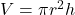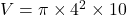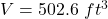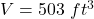## Initial Knowledge Check Question 2 The diameter of a cylindrical water tank is 8 ft, and its height is 10 ft. What i

Question

Initial Knowledge Check

Question 2

The diameter of a cylindrical water tank is 8 ft, and its height is 10 ft. What is the volume of the tank?

Use the value 3.14 for x, and round your answer to the nearest whole number.

St

D

ft

ft?

10 ft

5

?

in progress 0
5 months 2021-08-13T01:46:13+00:00 1 Answers 2 views 0

The volume of tank to the nearest cube is 503 ft³

Step-by-step explanation:

Given that,

Diameter of tank = 8 ft

Height = 10 ft

We need to calculate the volume of tank

Using formula of volume